# OOP in Action: The Samuelson Accelerator¶

Co-author: Natasha Watkins

## Paul Samuelson’s (1939) multiplier-accelerator model¶

This lecture creates nonstochastic and stochastic versions of Paul Samuelson’s celebrated multiplier accelerator model, as described in [Sam39]

Samuelson used a second-order linear difference equation to represent a model of national output based on three components:

• a national output identity asserting that national outcome is the sum of output devoted to consumption plus investment plus government purchases
• a Keynesian consumption function asserting that consumption at time $t$ is equal to a constant called the marginal propensity to consume times national output at time $t-1$
• an investment accelerator asserting that investment at time $t$ equals a constant called the accelerator coefficient times the difference in output between period $t-1$ and $t-2$
• the idea that consumption plus investment plus government purchases constitute aggregate demand that automatically call forth an equal amount of aggregate supply

(To read about linear difference equations see here or chapter IX of [Sar87])

Samuelson used the model to analyze how particular values of the marginal propensity to consume and the accelerator coefficient might give rise to transient business cycles in national output with alternative dynamic properties:

• smooth convergence to a constant level of output
• damped business cycles that eventually converge to a constant level of output
• persistent business cycles that neither dampen nor explode

Later in the lecture we present an extension to Samuelson’s model that simply adds a random shock to the right side of the national income identity (the random shock represents random fluctuations in aggregate demand

This modification makes national output become governed by a second-order stochastic linear difference equation that, with appropriate parameter values, gives rise to recurrent irregular business cycles.

(To read about stochastic linear difference equations see chapter XI of [Sar87])

### Structure of the model¶

In more detail, let’s assume that

• $\{G_t\}$ is a sequence of levels of government expenditures. We’ll start by setting $G_t = G \forall t$
• $\{C_t\}$ is a sequence of levels of aggregate consumption expenditures, a key endogenous variable in the model
• $\{I_t\}$ is a sequence of rates of investment, another key endogenous variable
• $\{Y_t\}$ is a sequence of levels of national income, yet another endogenous variable
• $a$ is the marginal propensity to consume in the Keynesian consumption function $C_t = a Y_{t-1} + \gamma$
• $b$ is the “accelerator coefficient” in the “investment accerator” $I_t = b (Y_{t-1} - Y_{t-2})$
• $\{\epsilon_{t}\}$ is an IID sequence standard normal random variables
• $\sigma \geq 0$ is a “volatility” parameter — setting $\sigma = 0$ recovers the nonstochastic case that we’ll start with

The model combines the consumption function

</tr></table>with the investment accelerator

 $$C_t = a Y_{t-1} + \gamma$$ (1)
</tr></table>and the national income identity

 $$I_t = b (Y_{t-1} - Y_{t-2})$$ (2)
</tr></table>- The parameter $a$ is peoples’ marginal propensity to consume out of income - equation (1) asserts that people consume a fraction of math:a in (0,1) of each additional dollar of income

• The parameter $b > 0$ is the investment accelerator coefficient - equation (2) asserts that people invest in physical capital when income is increasing and disinvest when it is decreasing

Equations (1), (2), and (3) imply the following second-order linear difference equation for national income:

 $$Y_t = C_t + I_t + G_t$$ (3)
</tr></table>or

 $$Y_t = (a+b) Y_{t-1} - b Y_{t-2} + (\gamma + G_t)$$
</tr></table>where $\rho_1 = (a+b)$ and $\rho_2 = -b$

To complete the model, we require two initial conditions

If the model is to generate time series for $t=0, \ldots, T$, we require initial values

 $$Y_t = \rho_1 Y_{t-1} + \rho_2 Y_{t-2} + (\gamma + G_t)$$ (4)
</tr></table>We’ll ordinarily set the parameters $(a,b)$ so that starting from an arbitrary pair of initial conditions $(\bar Y_{-1}, \bar Y_{-2})$, national income $Y_t$ converges to a constant value as $t$ becomes large

We are interested in studying

• the transient fluctuations in $Y_t$ as it converges to its steady state level
• the rate at which it converges to a steady state level

The deterministic version of the model described so far — meaning that no random shocks hit aggregate demand — has only transient fluctuations

We can convert the model to one that has persistent irregular fluctuations by adding a random shock to aggregate demand

### Stochastic version of the model¶

We create a random or stochastic version of the model by adding a random process of shocks or disturbances $\{\sigma \epsilon_t \}$ to the right side of equation (4), leading to the second-order scalar linear stochastic difference equation:

 $$Y_{-1} = \bar Y_{-1}, \quad Y_{-2} = \bar Y_{-2}$$
 $$Y_t = G_t + a (1-b) Y_{t-1} - a b Y_{t-2} + \sigma \epsilon_{t}$$ (5)

### Mathematical analysis of the model¶

To get started, let’s set $G_t \equiv 0$, $\sigma = 0$, and $\gamma = 0$.

Then we can write equation (5) as

</tr></table>or

 $$Y_t = \rho_1 Y_{t-1} + \rho_2 Y_{t-2}$$
</tr></table>To discover the properties of the solution of (6), it is useful first to form the characteristic polynomial for (6):

 $$Y_{t+2} - \rho_1 Y_{t+1} - \rho_2 Y_t = 0$$ (6)
</tr></table>where $z$ is possibly a complex number

We want to find the two zeros (a.k.a. roots) – namely $\lambda_1, \lambda_2$ – of the characteristic polynomial

These are two special values of $z$, say $z= \lambda_1$ and $z= \lambda_2$, such that if we set $z$ equal to one of these values in expresssion (7), the characteristic polynomial (7) equals zero:

 $$z^2 - \rho_1 z - \rho_2$$ (7)
</tr></table>Equation (8) is said to factor the characteristic polynomial

When the roots are complex, they will occur as a complex conjugate pair

When the roots are complex, it is convenient to represent them in the polar form

 $$z^2 - \rho_1 z - \rho_2 = (z- \lambda_1 ) (z -\lambda_2) = 0$$ (8)
</tr></table>where $r$ is the amplitude of the complex number and $\theta$ is its angle.

These can also be represented as

 $$\lambda_1 = r e^{i \theta}, \ \lambda_2 = r e^{-i \theta}$$
</tr></table>
 $$\lambda_1 = r (cos (\theta) + i \sin (\theta))$$

Given initial conditions $Y_{-1}, Y_{-2}$ we want to generate a solution of the difference equation (6)

It can be represented as

 $$\lambda_2 = r (cos (\theta) - i \sin(\theta))$$
</tr></table>where $c_1$ and $c_2$ are constants that depend on the two initial conditions and on $\rho_1, \rho_2$

When the roots are complex, some algebra that exploits the fact that the roots appear as a complex conjugate pair implies that

 $$Y_t = \lambda_1^t c_1 + \lambda_2^t c_2$$
</tr></table>where $\tilde c_1, \tilde c_2$ is a pair of constants chosen to satisfy the given initial conditions for $Y_{-1}, Y_{-2}$

This formula shows that when the roots are complex, $Y_t$ displays oscillations with period $\check p = \frac{2 \pi}{\theta}$ and damping factor $r$

Remark: Following [Sam39], we want to choose the parameters $a, b$ of the model so that the absolute values (of the possibly complex) roots $\lambda_1, \lambda_2$ of the characteristic polynomial are both strictly less than one:

 $$Y_t = \tilde c_1 r^t \cos(\theta t + \tilde c_1)$$
</tr></table>Remark: When both eigenvalues $\lambda_1, \lambda_2$ have absolute values strictly less than one, the absolute value of the larger one governs the rate of convergence to the steady state of the non stochastic version of the model

### Things this lecture does¶

1. Writes a function to generate simulations of a $\{Y_t\}$ sequence as a function of time. Say about 40 or 80 periods. The function checks that $a, b$ are set so that $\lambda\_1, \lambda\_2$ are less than unity in absolute value (also called “modulus”). The function also tells us whether the roots are complex, and, if they are complex, returns both their real and complex parts; if the roots are both real, the function returns their values. The function requires that we put in initial conditions for $Y_{-1}, Y_{-2}$
2. The lecture produces a version of a graph in chapter IX, p. 189, of [Sar87]. The graph maps values of $\rho_1, \rho_2$ into various regions – including ones that produce complex roots and therefore “cycles” in the outputs. We can use versions of this graph to help us guide how to set $a,b$
3. We use our function written to simulate paths that are stochastic (when $\sigma >0$)
4. We have written the function in a way that allows us to input $\{G_t\}$ paths of a few simple forms – e.g., one time jumps in $G$ at some time; and a permanent jump in $G$ that occurs at some time
5. We proceed to use the Samuelson multiplier-accererator model as a laboratory to make a simple OOP example that eventually can follow the example of the Solow model class in this lecture. One lesson from doing this is that in writing the function and class to simulate the model, the “state” that determines next period’s $Y_{t+1}$ is now not just the current value $Y_t$ but also the once lagged value $Y_{t-1}$. This involves a little more bookkeeping than is required in the Solow model class definition
6. We use the Samuelson multiplier-accelerator model as a vehicle for teaching how we can gradually add more and more fun features to the class. We want to have a method in the class that automatically generates a simulation, either nonstochastic ($\sigma=0$) or stochastic ($\sigma > 0$)
7. We also show how to map the Samuelson model into a simple instance of the LinearStateSpace class described here. We can use a LinearStateSpace instance to do various things that we did above with our homemade function and class. Among other things, we show by example that the eigenvalues of the matrix $A$ that we use to form the instance of the LinearStateSpace class for the Samuelson model equal the roots of the characteristic polynomial (7) for the Samuelson multiplier accelerator model. Here is the formula for the matrix $A$ in the linear state space system in the case that government expenditures are a constant $G$:
 $$| \lambda_j | < 1 \quad \quad \text{for } j = 1, 2$$
 $$A = \begin{bmatrix} 1 & 0 & 0 \cr \gamma + G & \rho_1 & \rho_2 \cr 0 & 1 & 0 \end{bmatrix}$$

## Let’s get to work¶

We’ll start by drawing an informative graph from page 189 of [Sar87]

In :
import numpy as np
import matplotlib.pyplot as plt

def param_plot():

"""this function creates the graph on page 189 of Sargent Macroeconomic Theory, second edition, 1987"""

fig, ax = plt.subplots(figsize=(12, 8))
ax.set_aspect('equal')

# Set axis
xmin, ymin = -3, -2
xmax, ymax = -xmin, -ymin
plt.axis([xmin, xmax, ymin, ymax])

# Set axis labels
ax.set(xticks=[], yticks=[])
ax.set_xlabel(r'$\rho_2$', fontsize=16)
ax.xaxis.set_label_position('top')
ax.set_ylabel(r'$\rho_1$', rotation=0, fontsize=16)
ax.yaxis.set_label_position('right')

# Draw (t1, t2) points
rho1 = np.linspace(-2, 2, 100)
ax.plot(rho1, -abs(rho1) + 1, c='black')
ax.plot(rho1, np.ones_like(rho1) * -1, c='black')
ax.plot(rho1, -(rho1**2 / 4), c='black')

# Turn normal axes off
for spine in ['left', 'bottom', 'top', 'right']:
ax.spines[spine].set_visible(False)

# Add arrows to represent axes
axes_arrows = {'arrowstyle': '<|-|>', 'lw': 1.3}
ax.annotate('', xy=(xmin, 0), xytext=(xmax, 0), arrowprops=axes_arrows)
ax.annotate('', xy=(0, ymin), xytext=(0, ymax), arrowprops=axes_arrows)

# Annotate the plot with equations
plot_arrowsl = {'arrowstyle': '-|>', 'connectionstyle': "arc3, rad=-0.2"}
plot_arrowsr = {'arrowstyle': '-|>', 'connectionstyle': "arc3, rad=0.2"}
ax.annotate(r'$\rho_1 + \rho_2 < 1$', xy=(0.5, 0.3), xytext=(0.8, 0.6),
arrowprops=plot_arrowsr, fontsize='12')
ax.annotate(r'$\rho_1 + \rho_2 = 1$', xy=(0.38, 0.6), xytext=(0.6, 0.8),
arrowprops=plot_arrowsr, fontsize='12')
ax.annotate(r'$\rho_2 < 1 + \rho_1$', xy=(-0.5, 0.3), xytext=(-1.3, 0.6),
arrowprops=plot_arrowsl, fontsize='12')
ax.annotate(r'$\rho_2 = 1 + \rho_1$', xy=(-0.38, 0.6), xytext=(-1, 0.8),
arrowprops=plot_arrowsl, fontsize='12')
ax.annotate(r'$\rho_2 = -1$', xy=(1.5, -1), xytext=(1.8, -1.3),
arrowprops=plot_arrowsl, fontsize='12')
ax.annotate(r'${\rho_1}^2 + 4\rho_2 = 0$', xy=(1.15, -0.35),
xytext=(1.5, -0.3), arrowprops=plot_arrowsr, fontsize='12')
ax.annotate(r'${\rho_1}^2 + 4\rho_2 < 0$', xy=(1.4, -0.7),
xytext=(1.8, -0.6), arrowprops=plot_arrowsr, fontsize='12')

# Label categories of solutions
ax.text(1.5, 1, 'Explosive\n growth', ha='center', fontsize=16)
ax.text(-1.5, 1, 'Explosive\n oscillations', ha='center', fontsize=16)
ax.text(0.05, -1.5, 'Explosive oscillations', ha='center', fontsize=16)
ax.text(0.09, -0.5, 'Damped oscillations', ha='center', fontsize=16)

# Add small marker to y-axis
ax.axhline(y=1.005, xmin=0.495, xmax=0.505, c='black')
ax.text(-0.12, -1.12, '-1', fontsize=10)
ax.text(-0.12, 0.98, '1', fontsize=10)

return fig

param_plot()
plt.show()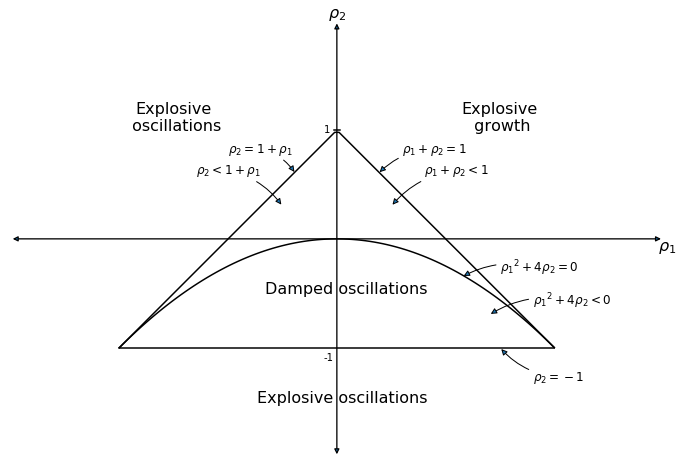### Explanation of the graph¶

The graph portrays regions in which the $(\lambda_1, \lambda_2)$ root pairs implied by the $(\rho_1 = (a+b), \rho_2 = - b)$ difference equation parameter pairs in the Samuelson model are such that:

• $(\lambda_1, \lambda_2)$ are complex with modulus less than $1$ - in this case, the $\{Y_t\}$ sequence displays damped oscillations
• $(\lambda_1, \lambda_2)$ are both real, but one is strictly greater than $1$ - this leads to explosive growth
• $(\lambda_1, \lambda_2)$ are both real, but one is strictly less than $-1$ - this leads to explosive oscillations
• $(\lambda_1, \lambda_2)$ are both real and both are less than $1$ in absolute value - in this case, there is smooth convergence to the steady state without damped cycles

Later we’ll present the graph with a red mark showing the particular point implied by the setting of $(a,b)$

### Function to describe implications of characteristic polynomial¶

In :
def categorize_solution(rho1, rho2):
"""this function takes values of rho1 and rho2 and uses them to classify the type of solution"""

discriminant = rho1 ** 2 + 4 * rho2
if rho2 > 1 + rho1 or rho2 < -1:
print('Explosive oscillations')
elif rho1 + rho2 > 1:
print('Explosive growth')
elif discriminant < 0:
print('Roots are complex with modulus less than one; therefore damped oscillations')
else:
print('Roots are real and absolute values are less than zero; therfore get smooth convergence to a steady state')

In :
### Test the categorize_solution function

categorize_solution(1.3, -.4)

Roots are real and absolute values are less than zero; therfore get smooth convergence to a steady state

none
Roots are real and absolute values are less than zero; therfore get smooth convergence to a steady state

### Function for plotting $Y_t$ paths¶

A useful function for our work below

In :
def plot_y(function=None):
"""function plots path of Y_t"""
plt.subplots(figsize=(12, 8))
plt.plot(function)
plt.xlabel('Time $t$')
plt.ylabel('$Y_t$', rotation=0)
plt.grid()
plt.show()


### Manual or “by hand” root calculations¶

The following function calculates roots of the characteristic polynomial using high school algebra

(We’ll calculate the roots in other ways later)

The function also plots a $Y_t$ starting from initial conditions that we set

In :
from cmath import sqrt

##=== This is a 'manual' method ===#

def y_nonstochastic(y_0=100, y_1=80, alpha=.92, beta=.5, gamma=10, n=80):

"""Takes values of parameters and computes roots of characteristic polynomial.
It tells whether they are real or complex and whether they are less than unity in absolute value.
It also computes a simulation of length n starting from the two given initial conditions for national income"""

roots = []

rho1 = alpha + beta
rho2 = -beta

print('rho_1 is ', rho1)
print('rho_2 is ', rho2)

discriminant = rho1 ** 2 + 4 * rho2

if discriminant == 0:
roots.append(-rho1 / 2)
print('Single real root: ')
print(''.join(str(roots)))
elif discriminant > 0:
roots.append((-rho1 + sqrt(discriminant).real) / 2)
roots.append((-rho1 - sqrt(discriminant).real) / 2)
print('Two real roots: ')
print(''.join(str(roots)))
else:
roots.append((-rho1 + sqrt(discriminant)) / 2)
roots.append((-rho1 - sqrt(discriminant)) / 2)
print('Two complex roots: ')
print(''.join(str(roots)))

if all(abs(root) < 1 for root in roots):
print('Absolute values of roots are less than one')
else:
print('Absolute values of roots are not less than one')

def transition(x, t): return rho1 * x[t - 1] + rho2 * x[t - 2] + gamma

y_t = [y_0, y_1]

for t in range(2, n):
y_t.append(transition(y_t, t))

return y_t

plot_y(y_nonstochastic())

rho_1 is  1.42
rho_2 is  -0.5
Two real roots:
[-0.6459687576256715, -0.7740312423743284]
Absolute values of roots are less than one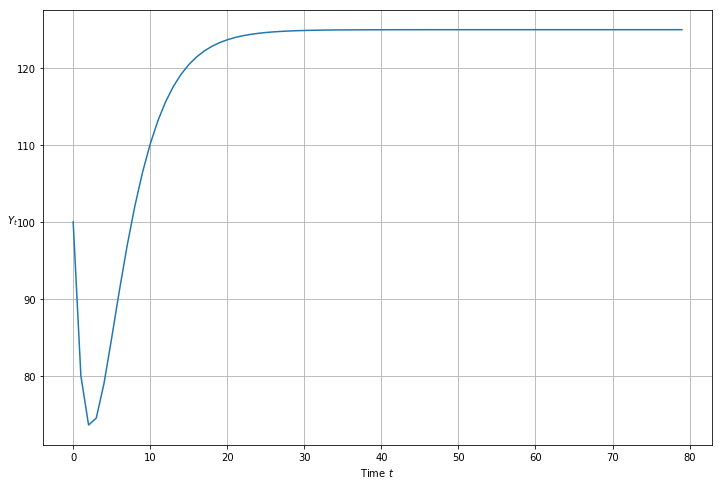none
rho_1 is  1.42
rho_2 is  -0.5
Two real roots:
[-0.6459687576256715, -0.7740312423743284]
Absolute values of roots are less than one### Reverse engineering parameters to generate damped cycles¶

The next cell writes code that takes as inputs the modulus $r$ and phase $\phi$ of a conjugate pair of complex numbers in polar form

</tr></table>- The code assumes that these two complex numbers are the roots of the characteristic polynomial

• It then reverse engineers $(a,b)$ and $(\rho_1, \rho_2)$, pairs that would generate those roots
In :
### code to reverse engineer a  cycle
### y_t = r^t (c_1 cos(phi t) + c2 sin(phi t))
###

import cmath
import math

def f(r, phi):
"""
Takes modulus r and angle phi of complex number r exp(j phi)
and creates rho1 and rho2 of characteristic polynomial for which
r exp(j phi) and r exp(- j phi) are complex roots.

Returns the multiplier coefficient a and the accelerator coefficient b
that verifies those roots.
"""
g1 = cmath.rect(r, phi)  # Generate two complex roots
g2 = cmath.rect(r, -phi)
rho1 = g1 + g2           # Implied rho1, rho2
rho2 = -g1 * g2
b = -rho2                # Reverse engineer a and b that validate these
a = rho1 - b
return rho1, rho2, a, b

## Now let's use the function in an example
## Here are the example paramters

r = .95
period = 10   # Length of cycle in units of time
phi = 2 * math.pi/period

## Apply the function

rho1, rho2, a, b = f(r, phi)

print("a, b = ", a, b)
print("rho1, rho2 =", rho1, rho2)

a, b =  (0.6346322893124001+0j) (0.9024999999999999-0j)
rho1, rho2 = (1.5371322893124+0j) (-0.9024999999999999+0j)

none
a, b =  (0.6346322893124001+0j) (0.9024999999999999-0j)
rho1, rho2 = (1.5371322893124+0j) (-0.9024999999999999+0j)
In :
## Print the real components of rho1 and rho2

rho1 = rho1.real
rho2 = rho2.real

rho1, rho2

Out:
$$\left ( 1.5371322893124, \quad -0.9024999999999999\right )$$
none
(1.5371322893124, -0.9024999999999999)

### Root finding using numpy¶

Here we’ll use numpy to compute the roots of the characteristic polynomial

In :
r1, r2 = np.roots([1, -rho1, -rho2])

p1 = cmath.polar(r1)
p2 = cmath.polar(r2)

print("r, phi =", r, phi)
print("p1, p2 = ", p1, p2)
# print("g1, g2 = ", g1, g2)

print("a, b =", a, b)
print("rho1, rho2 =", rho1, rho2)

r, phi = 0.95 0.6283185307179586
p1, p2 =  (0.95, 0.6283185307179586) (0.95, -0.6283185307179586)
a, b = (0.6346322893124001+0j) (0.9024999999999999-0j)
rho1, rho2 = 1.5371322893124 -0.9024999999999999

none
r, phi = 0.95 0.6283185307179586
p1, p2 =  (0.95, 0.6283185307179586) (0.95, -0.6283185307179586)
a, b = (0.6346322893124001+0j) (0.9024999999999999-0j)
rho1, rho2 = 1.5371322893124 -0.9024999999999999
In :
##=== This method uses numpy to calculate roots ===#

def y_nonstochastic(y_0=100, y_1=80, alpha=.9, beta=.8, gamma=10, n=80):

""" Rather than computing the roots of the characteristic polynomial by hand as we did earlier, this function
enlists numpy to do the work for us """

# Useful constants
rho1 = alpha + beta
rho2 = -beta

categorize_solution(rho1, rho2)

# Find roots of polynomial
roots = np.roots([1, -rho1, -rho2])
print('Roots are', roots)

# Check if real or complex
if all(isinstance(root, complex) for root in roots):
print('Roots are complex')
else:
print('Roots are real')

# Check if roots are less than one
if all(abs(root) < 1 for root in roots):
print('Roots are less than one')
else:
print('Roots are not less than one')

# Define transition equation
def transition(x, t): return rho1 * x[t - 1] + rho2 * x[t - 2] + gamma

# Set initial conditions
y_t = [y_0, y_1]

# Generate y_t series
for t in range(2, n):
y_t.append(transition(y_t, t))

return y_t

plot_y(y_nonstochastic())

Roots are complex with modulus less than one; therefore damped oscillations
Roots are [ 0.85+0.27838822j  0.85-0.27838822j]
Roots are complex
Roots are less than one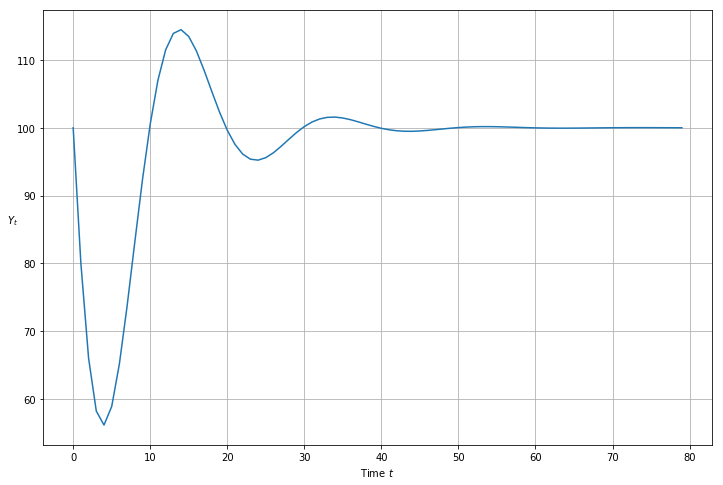none
Roots are complex with modulus less than one; therefore damped oscillations
Roots are [ 0.85+0.27838822j  0.85-0.27838822j]
Roots are complex
Roots are less than one### Reverse engineered complex roots: example¶

The next cell studies the implications of revese engineered complex roots

We’ll generate an undamped cycle of period 10

In :
r = 1   # generates undamped, nonexplosive cycles

period = 10   #  length of cycle in units of time
phi = 2 * math.pi/period

## Apply the reverse engineering function f

rho1, rho2, a, b = f(r, phi)

a = a.real  # drop the imaginary part so that it is a valid input into y_nonstochastic
b = b.real

print("a, b =", a, b)

ytemp = y_nonstochastic(alpha=a, beta=b, y_0=20, y_1=30)
plot_y(ytemp)

a, b = 0.6180339887498949 1.0
Roots are complex with modulus less than one; therefore damped oscillations
Roots are [ 0.80901699+0.58778525j  0.80901699-0.58778525j]
Roots are complex
Roots are less than one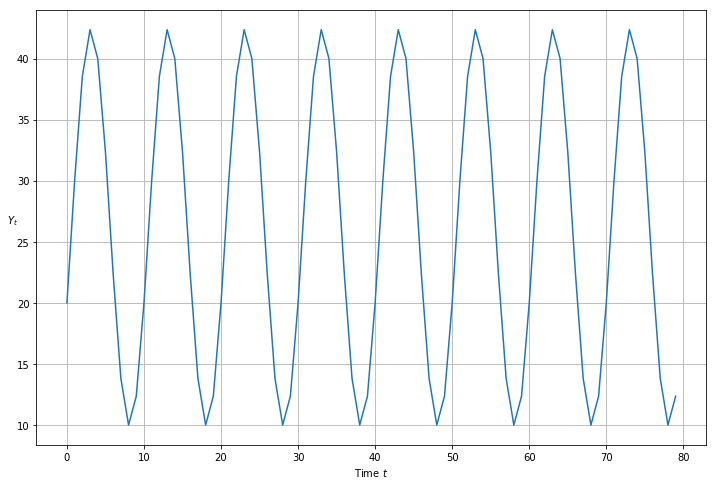none
a, b = 0.6180339887498949 1.0
Roots are complex with modulus less than one; therefore damped oscillations
Roots are [ 0.80901699+0.58778525j  0.80901699-0.58778525j]
Roots are complex
Roots are less than one### Interactive demonstration¶

We'll use some widgets to show some of the consequences of parameters $(a,b)$ that are associated with complex conjugate roots of the characteristic polynomial. The widget will allow us to specify the amplitude and angle of the complex roots of the characteristic polynomial. (It will reverse engineer the $(a,b)$ that imply those roots.)

In :
from ipywidgets import interact

def choose_r_phi(r, phi):

rho1, rho2, a, b = f(r, phi)
a = a.real  # drop the imaginary part so that it is a valid input into y_nonstochastic
b = b.real
ytemp = y_nonstochastic(alpha=a, beta=b, y_0=20, y_1=30)

return plot_y(ytemp)

In :
periodhighest = 20

interact(choose_r_phi, r=(0, 1.5), phi=(math.pi/periodhighest, 10 * math.pi/periodhighest))

Out:
<function __main__.choose_r_phi>

### Digression: using sympy to find roots¶

We can also use sympy to compute analytic formulas for the roots

In :
import sympy
from sympy import Symbol, init_printing
init_printing()

r1 = Symbol("rho_1")
r2 = Symbol("rho_2")
z = Symbol("z")

sympy.solve(z**2 - r1*z - r2, z)

Out:
$$\left [ \frac{\rho_{1}}{2} - \frac{1}{2} \sqrt{\rho_{1}^{2} + 4 \rho_{2}}, \quad \frac{\rho_{1}}{2} + \frac{1}{2} \sqrt{\rho_{1}^{2} + 4 \rho_{2}}\right ]$$
 $$\lambda_1 = r \exp(i \phi), \quad \lambda_2 = r \exp(- i \phi)$$
 $$\left [ \frac{\rho_{1}}{2} - \frac{1}{2} \sqrt{\rho_{1}^{2} + 4 \rho_{2}}, \quad \frac{\rho_{1}}{2} + \frac{1}{2} \sqrt{\rho_{1}^{2} + 4 \rho_{2}}\right ]$$
In :
a = Symbol("alpha")
b = Symbol("beta")
r1 = a + b
r2 = -b

sympy.solve(z**2 - r1*z - r2, z)

Out:
$$\left [ \frac{\alpha}{2} + \frac{\beta}{2} - \frac{1}{2} \sqrt{\alpha^{2} + 2 \alpha \beta + \beta^{2} - 4 \beta}, \quad \frac{\alpha}{2} + \frac{\beta}{2} + \frac{1}{2} \sqrt{\alpha^{2} + 2 \alpha \beta + \beta^{2} - 4 \beta}\right ]$$
 $$\left [ \frac{\alpha}{2} + \frac{\beta}{2} - \frac{1}{2} \sqrt{\alpha^{2} + 2 \alpha \beta + \beta^{2} - 4 \beta}, \quad \frac{\alpha}{2} + \frac{\beta}{2} + \frac{1}{2} \sqrt{\alpha^{2} + 2 \alpha \beta + \beta^{2} - 4 \beta}\right ]$$

## Stochastic shocks¶

Now we’ll construct some code to simulate the stochastic version of the model that emerges when we add a random shock process to aggregate demand

In :
def y_stochastic(y_0=0, y_1=0, alpha=0.8, beta=0.2, gamma=10, n=100, sigma=5):

"""This function takes parameters of a stochastic version of the model and proceeds to analyze
the roots of the characteristic polynomial and also generate a simulation"""

# Useful constants
rho1 = alpha + beta
rho2 = -beta

# Categorize solution
categorize_solution(rho1, rho2)

# Find roots of polynomial
roots = np.roots([1, -rho1, -rho2])
print(roots)

# Check if real or complex
if all(isinstance(root, complex) for root in roots):
print('Roots are complex')
else:
print('Roots are real')

# Check if roots are less than one
if all(abs(root) < 1 for root in roots):
print('Roots are less than one')
else:
print('Roots are not less than one')

# Generate shocks
epsilon = np.random.normal(0, 1, n)

# Define transition equation
def transition(x, t): return rho1 * \
x[t - 1] + rho2 * x[t - 2] + gamma + sigma * epsilon[t]

# Set initial conditions
y_t = [y_0, y_1]

# Generate y_t series
for t in range(2, n):
y_t.append(transition(y_t, t))

return y_t

plot_y(y_stochastic())

Roots are real and absolute values are less than zero; therfore get smooth convergence to a steady state
[ 0.7236068  0.2763932]
Roots are real
Roots are less than one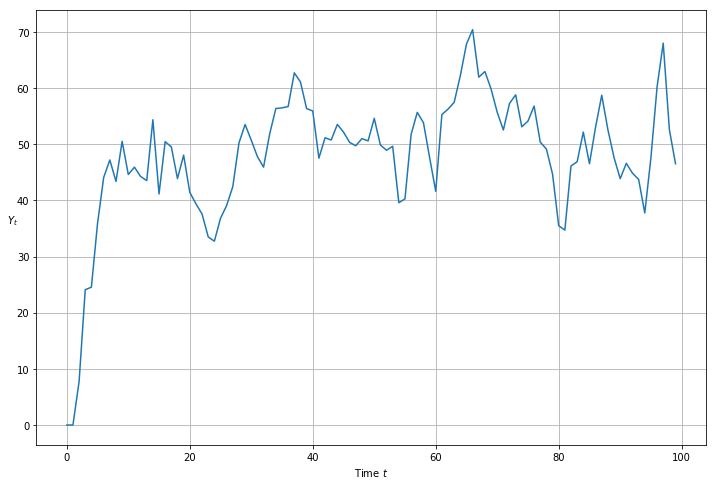none
Roots are real and absolute values are less than zero; therfore get smooth convergence to a steady state
[ 0.7236068  0.2763932]
Roots are real
Roots are less than oneLet’s do a simulation in which there are shocks and the characteristic polynomial has complex roots

In :
r = .97

period = 10   #  length of cycle in units of time
phi = 2 * math.pi/period

### apply the  reverse engineering function f

rho1, rho2, a, b = f(r, phi)

a = a.real  # drop the imaginary part so that it is a valid input into y_nonstochastic
b = b.real

print("a, b = ", a, b)
plot_y(y_stochastic(y_0=40, y_1 = 42, alpha=a, beta=b, sigma=2, n=100))

a, b =  0.6285929690873979 0.9409000000000001
Roots are complex with modulus less than one; therefore damped oscillations
[ 0.78474648+0.57015169j  0.78474648-0.57015169j]
Roots are complex
Roots are less than one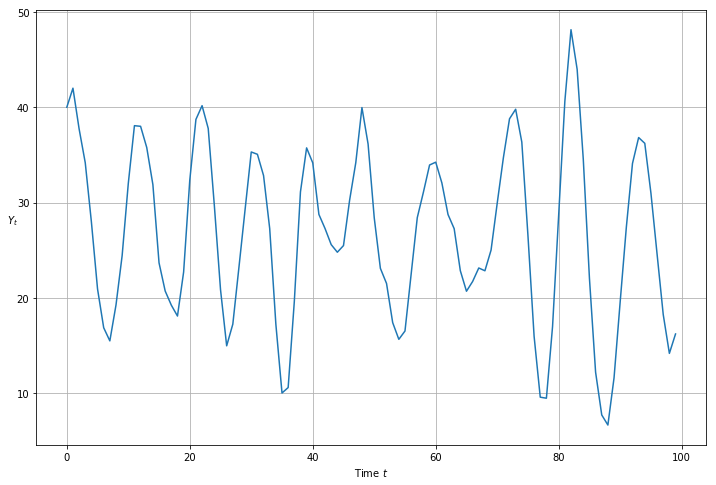none
a, b =  0.6285929690873979 0.9409000000000001
Roots are complex with modulus less than one; therefore damped oscillations
[ 0.78474648+0.57015169j  0.78474648-0.57015169j]
Roots are complex
Roots are less than one## Government spending¶

This function computes a response to either a permanent or one-off increase in government expenditures

In :
def y_stochastic_g(y_0=20,
y_1=20,
alpha=0.8,
beta=0.2,
gamma=10,
n=100,
sigma=2,
g=0,
g_t=0,
duration='permanent'):

"""This program computes a response to a permanent increase in government expenditures that occurs
at time 20"""

# Useful constants
rho1 = alpha + beta
rho2 = -beta

# Categorize solution
categorize_solution(rho1, rho2)

# Find roots of polynomial
roots = np.roots([1, -rho1, -rho2])
print(roots)

# Check if real or complex
if all(isinstance(root, complex) for root in roots):
print('Roots are complex')
else:
print('Roots are real')

# Check if roots are less than one
if all(abs(root) < 1 for root in roots):
print('Roots are less than one')
else:
print('Roots are not less than one')

# Generate shocks
epsilon = np.random.normal(0, 1, n)

def transition(x, t, g):

# Non-stochastic - separated to avoid generating random series when not needed
if sigma == 0:
return rho1 * x[t - 1] + rho2 * x[t - 2] + gamma + g

# Stochastic
else:
epsilon = np.random.normal(0, 1, n)
return rho1 * x[t - 1] + rho2 * x[t - 2] + gamma + g + sigma * epsilon[t]

# Create list and set initial conditions
y_t = [y_0, y_1]

# Generate y_t series
for t in range(2, n):

# No government spending
if g == 0:
y_t.append(transition(y_t, t))

# Government spending (no shock)
elif g != 0 and duration == None:
y_t.append(transition(y_t, t))

# Permanent government spending shock
elif duration == 'permanent':
if t < g_t:
y_t.append(transition(y_t, t, g=0))
else:
y_t.append(transition(y_t, t, g=g))

# One-off government spending shock
elif duration == 'one-off':
if t == g_t:
y_t.append(transition(y_t, t, g=g))
else:
y_t.append(transition(y_t, t, g=0))
return y_t


A permanent government spending shock can be simulated as follows

In :
plot_y(y_stochastic_g(g=10, g_t=20, duration='permanent'))

Roots are real and absolute values are less than zero; therfore get smooth convergence to a steady state
[ 0.7236068  0.2763932]
Roots are real
Roots are less than one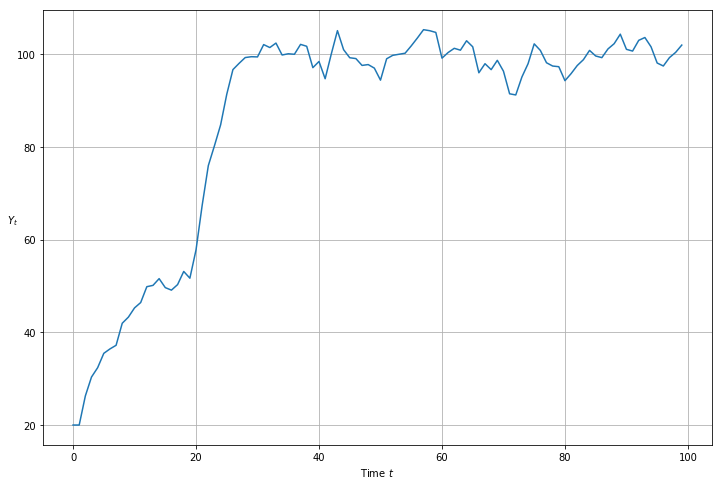none
Roots are real and absolute values are less than zero; therfore get smooth convergence to a steady state
[ 0.7236068  0.2763932]
Roots are real
Roots are less than oneWe can also see the response to a one time jump in government expenditures

In :
plot_y(y_stochastic_g(g=500, g_t=50, duration='one-off'))

Roots are real and absolute values are less than zero; therfore get smooth convergence to a steady state
[ 0.7236068  0.2763932]
Roots are real
Roots are less than one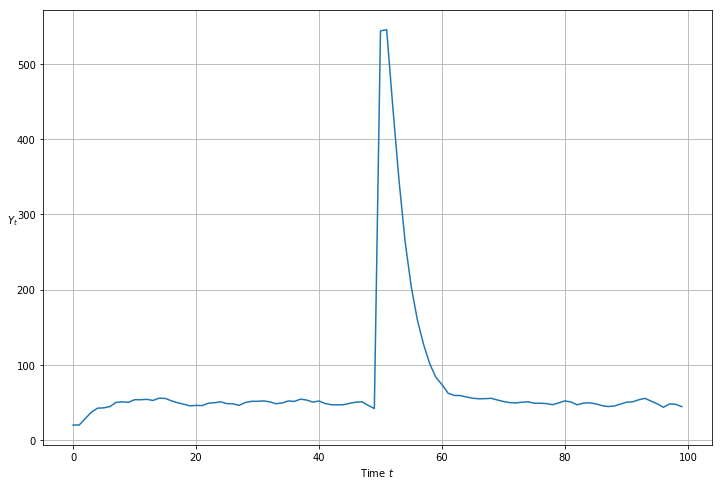none
Roots are real and absolute values are less than zero; therfore get smooth convergence to a steady state
[ 0.7236068  0.2763932]
Roots are real
Roots are less than one## Wrapping everything into a class¶

Up to now we have written functions to do the work

Now we’ll roll up our sleeves and write a Python class called Samuelson for the Samuleson model

In :
class Samuelson():

r"""This class represents the Samuelson model, otherwise known as the
multiple-accelerator model. The model combines the Keynesian multipler
with the accelerator theory of investment.

The path of output is governed by a linear second-order difference equation

.. math::

Y_t =  + \alpha (1 + \beta) Y_{t-1} - \alpha \beta Y_{t-2}

Parameters
----------
y_0 : scalar
Initial condition for Y_0
y_1 : scalar
Initial condition for Y_1
alpha : scalar
Marginal propensity to consume
beta : scalar
Accelerator coefficient
n : int
Number of iterations
sigma : scalar
Volatility parameter. Must be greater than or equal to 0. Set
equal to 0 for non-stochastic model.
g : scalar
Government spending shock
g_t : int
Time at which government spending shock occurs. Must be specified
when duration != None.
duration : {None, 'permanent', 'one-off'}
Specifies type of government spending shock. If none, government
spending equal to g for all t.

"""

def __init__(self,
y_0=100,
y_1=50,
alpha=1.3,
beta=0.2,
gamma=10,
n=100,
sigma=0,
g=0,
g_t=0,
duration=None):

self.y_0, self.y_1, self.alpha, self.beta = y_0, y_1, alpha, beta
self.n, self.g, self.g_t, self.duration = n, g, g_t, duration
self.gamma, self.sigma = gamma, sigma
self.rho1 = alpha + beta
self.rho2 = -beta
self.roots = np.roots([1, -self.rho1, -self.rho2])

def root_type(self):
if all(isinstance(root, complex) for root in self.roots):
return 'Complex conjugate'
elif len(self.roots) > 1:
return 'Double real'
else:
return 'Single real'

def root_less_than_one(self):
if all(abs(root) < 1 for root in self.roots):
return True

def solution_type(self):
rho1, rho2 = self.rho1, self.rho2
discriminant = rho1 ** 2 + 4 * rho2
if rho2 >= 1 + rho1 or rho2 <= -1:
return 'Explosive oscillations'
elif rho1 + rho2 >= 1:
return 'Explosive growth'
elif discriminant < 0:
return 'Damped oscillations'
else:

def _transition(self, x, t, g):

# Non-stochastic - separated to avoid generating random series when not needed
if self.sigma == 0:
return self.rho1 * x[t - 1] + self.rho2 * x[t - 2] + self.gamma + g

# Stochastic
else:
epsilon = np.random.normal(0, 1, self.n)
return self.rho1 * x[t - 1] + self.rho2 * x[t - 2] + self.gamma + g + self.sigma * epsilon[t]

def generate_series(self):

# Create list and set initial conditions
y_t = [self.y_0, self.y_1]

# Generate y_t series
for t in range(2, self.n):

# No government spending
if self.g == 0:
y_t.append(self._transition(y_t, t))

# Government spending (no shock)
elif self.g != 0 and self.duration == None:
y_t.append(self._transition(y_t, t))

# Permanent government spending shock
elif self.duration == 'permanent':
if t < self.g_t:
y_t.append(self._transition(y_t, t, g=0))
else:
y_t.append(self._transition(y_t, t, g=self.g))

# One-off government spending shock
elif self.duration == 'one-off':
if t == self.g_t:
y_t.append(self._transition(y_t, t, g=self.g))
else:
y_t.append(self._transition(y_t, t, g=0))
return y_t

def summary(self):
print('Summary\n' + '-' * 50)
print('Root type: ' + self.root_type())
print('Solution type: ' + self.solution_type())
print('Roots: ' + str(self.roots))

if self.root_less_than_one() == True:
print('Absolute value of roots is less than one')
else:
print('Absolute value of roots is not less than one')

if self.sigma > 0:
print('Stochastic series with sigma = ' + str(self.sigma))
else:
print('Non-stochastic series')

if self.g != 0:
print('Government spending equal to ' + str(self.g))

if self.duration != None:
print(self.duration.capitalize() +
' government spending shock at t = ' + str(self.g_t))

def plot(self):
fig, ax = plt.subplots(figsize=(12, 8))
ax.plot(self.generate_series())
ax.set(xlabel='Iteration', xlim=(0, self.n))
ax.set_ylabel('$Y_t$', rotation=0)
ax.grid()

# Add parameter values to plot
paramstr = '$\\alpha=%.2f$\n$\\beta=%.2f$\n$\\gamma=%.2f$\n$\\sigma=%.2f$\n$\\rho_1=%.2f$\n$\\rho_2=%.2f$'%(
self.alpha, self.beta, self.gamma, self.sigma, self.rho1, self.rho2)
ax.text(0.87, 0.05, paramstr, transform=ax.transAxes,
fontsize=12, bbox=props, va='bottom')

return fig

def param_plot(self):

# Uses the param_plot() function defined earlier (it is then able
# to be used standalone or as part of the model)

fig = param_plot()
ax = fig.gca()

# Add lambda values to legend
for i, root in enumerate(self.roots):
if isinstance(root, complex):
operator = ['+', ''] # Need to fill operator for positive as string is split apart
label = r'$\lambda_{0} = {1.real:.2f} {2} {1.imag:.2f}i$'.format(i+1, sam.roots[i], operator[i])
else:
label = r'$\lambda_{0} = {1.real:.2f}$'.format(i+1, sam.roots[i])
ax.scatter(0, 0, 0, label=label) # dummy to add to legend

# Add rho pair to plot
ax.scatter(self.rho1, self.rho2, 100, 'red', '+', label=r'$(\ \rho_1, \ \rho_2 \ )$', zorder=5)

plt.legend(fontsize=12, loc=3)

return fig


### Illustration of Samuelson class¶

Now we’ll put our Samuelson class to work on an example

In :
sam = Samuelson(alpha=0.8, beta=0.5, sigma=2, g=10, g_t=20, duration='permanent')
sam.summary()

Summary
--------------------------------------------------
Root type: Complex conjugate
Solution type: Damped oscillations
Roots: [ 0.65+0.27838822j  0.65-0.27838822j]
Absolute value of roots is less than one
Stochastic series with sigma = 2
Government spending equal to 10
Permanent government spending shock at t = 20

none
Summary
---------------------------------------------------------
Root type: Complex conjugate
Solution type: Damped oscillations
Roots: [ 0.65+0.27838822j  0.65-0.27838822j]
Absolute value of roots is less than one
Stochastic series with sigma = 2
Government spending equal to 10
Permanent government spending shock at t = 20
In :
sam.plot()
plt.show()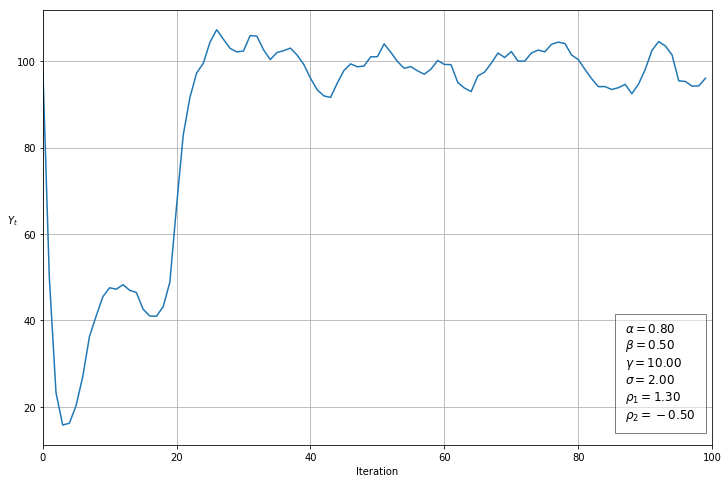### Using the graph¶

We’ll use our graph to show where the roots lie and how their location is consistent with the behavior of the path just graphed

The red $+$ sign shows the location of the roots

In :
sam.param_plot()
plt.show()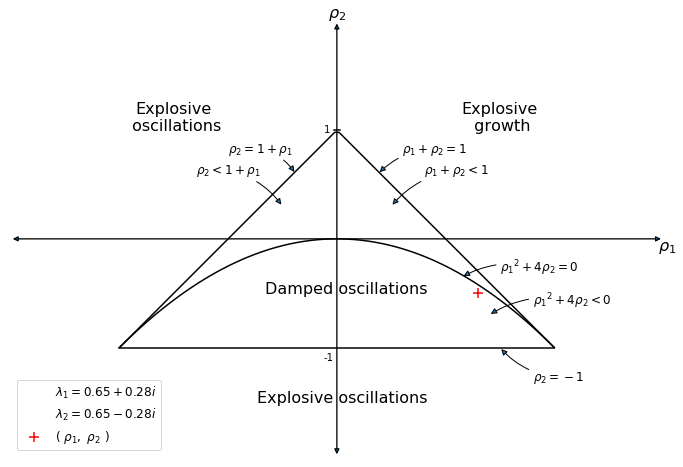## Using the LinearStateSpace class¶

It turns out that we can use the QuantEcon.py LinearStateSpace class to do much of the work that we have done from scratch above

Here is how we map the Samuelson model into an instance of a LinearStateSpace class

In :
from quantecon import LinearStateSpace

""" This script maps the Samuelson model in the the LinearStateSpace class"""
alpha = 0.8
beta = 0.9
rho1 = alpha + beta
rho2 = -beta
gamma = 10
sigma = 1
g = 10
n = 100

A = [[1,        0,        0],
[gamma + g, rho1, rho2],
[0,        1,        0]]

G = [[gamma + g, rho1, rho2],         # this is Y_{t+1}
[gamma,    alpha,    0],         # this is C_{t+1}
[0,     beta,    -beta]]         # this is I_{t+1}

mu_0 = [1, 100, 100]
C = np.zeros((3,1))
C = sigma # stochastic

sam_t = LinearStateSpace(A, C, G, mu_0=mu_0)

x, y = sam_t.simulate(ts_length=n)

fig, axes = plt.subplots(3, 1, sharex=True, figsize=(15, 8))
titles = ['Output ($Y_t$)', 'Consumption ($C_t$)', 'Investment ($I_t$)']
colors = ['darkblue', 'red', 'purple']
for ax, series, title, color in zip(axes, y, titles, colors):
ax.plot(series, color=color)
ax.set(title=title, xlim=(0, n))
ax.grid()

axes[-1].set_xlabel('Iteration')

plt.show()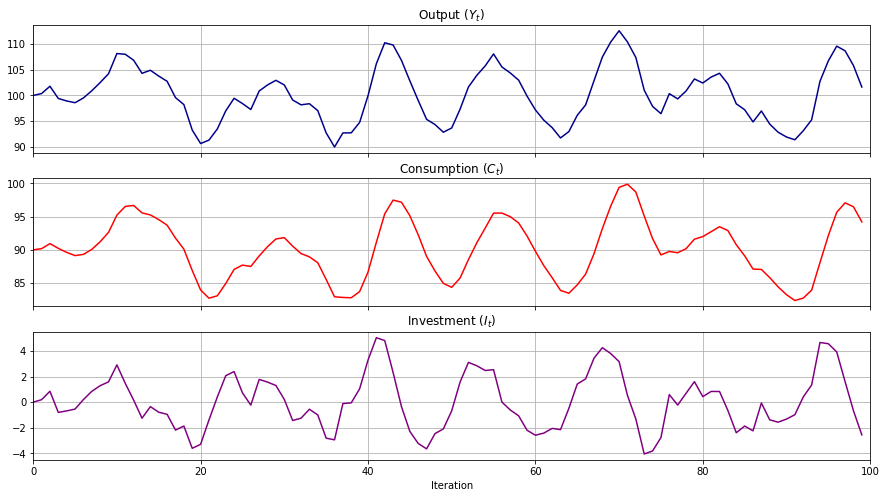### Other methods in the LinearStateSpace class¶

Let’s plot impulse response functions for the instance of the Samuelson model using a method in the LinearStateSpace class

In :
imres = sam_t.impulse_response()
imres = np.asarray(imres)
y1 = imres[:, :, 0]
y2 = imres[:, :, 1]
y1.shape

Out:
$$\left ( 2, \quad 6, \quad 1\right )$$

</tr></table>Now let’s compute the zeros of the characteristic polynomial by simply calculating the eigenvalues of $A$

In :
A = np.asarray(A)
w, v = np.linalg.eig(A)
print(w)

[ 0.85+0.42130749j  0.85-0.42130749j  1.00+0.j        ]

none
[ 0.85+0.42130749j  0.85-0.42130749j  1.00+0.j        ]

### Inheriting methods from LinearStateSpace¶

We could also create a subclass of LinearStateSpace (inheriting all its methods and attributes) to add more functions to use

In :
class SamuelsonLSS(LinearStateSpace):

"""
this subclass creates a Samuelson multiplier-accelerator model
as a linear state space system
"""
def __init__(self,
y_0=100,
y_1=100,
alpha=0.8,
beta=0.9,
gamma=10,
sigma=1,
g=10):

self.alpha, self.beta = alpha, beta
self.y_0, self.y_1, self.g = y_0, y_1, g
self.gamma, self.sigma = gamma, sigma

# Define intial conditions
self.mu_0 = [1, y_0, y_1]

self.rho1 = alpha + beta
self.rho2 = -beta

# Define transition matrix
self.A = [[1,                 0,         0],
[gamma + g, self.rho1, self.rho2],
[0,                 1,         0]]

# Define output matrix
self.G = [[gamma + g, self.rho1, self.rho2],         # this is Y_{t+1}
[gamma,         alpha,         0],         # this is C_{t+1}
[0,              beta,     -beta]]         # this is I_{t+1}

self.C = np.zeros((3, 1))
self.C = sigma  # stochastic

# Initialize LSS with parameters from Samuleson model
LinearStateSpace.__init__(self, self.A, self.C, self.G, mu_0=self.mu_0)

def plot_simulation(self, ts_length=100, stationary=True):

# Temporarily store original parameters
temp_mu = self.mu_0
temp_Sigma = self.Sigma_0

# Set distribution parameters equal to their stationary values for simulation
if stationary == True:
try:
self.mu_x, self.mu_y, self.sigma_x, self.sigma_y = self.stationary_distributions()
self.mu_0 = self.mu_y
self.Sigma_0 = self.sigma_y
# Exception where no convergence achieved when calculating stationary distributions
except ValueError:
print('Stationary distribution does not exist')

x, y = self.simulate(ts_length)

fig, axes = plt.subplots(3, 1, sharex=True, figsize=(15, 8))
titles = ['Output ($Y_t$)', 'Consumption ($C_t$)', 'Investment ($I_t$)']
colors = ['darkblue', 'red', 'purple']
for ax, series, title, color in zip(axes, y, titles, colors):
ax.plot(series, color=color)
ax.set(title=title, xlim=(0, n))
ax.grid()

axes[-1].set_xlabel('Iteration')

# Reset distribution parameters to their initial values
self.mu_0 = temp_mu
self.Sigma_0 = temp_Sigma

return fig

def plot_irf(self, j=5):

x, y = self.impulse_response(j)

# Reshape into 3 x j matrix for plotting purposes
yimf = np.array(y).flatten().reshape(j+1, 3).T

fig, axes = plt.subplots(3, 1, sharex=True, figsize=(15, 8))
labels = ['$Y_t$', '$C_t$', '$I_t$']
colors = ['darkblue', 'red', 'purple']
for ax, series, label, color in zip(axes, yimf, labels, colors):
ax.plot(series, color=color)
ax.set(xlim=(0, j))
ax.grid()

axes.set_title('Impulse Response Functions')
axes[-1].set_xlabel('Iteration')

return fig

def multipliers(self, j=5):
x, y = self.impulse_response(j)
return np.sum(np.array(y).flatten().reshape(j+1, 3), axis=0)


### Illustrations¶

Let’s show how we can use the SamuelsonLSS

In :
samlss = SamuelsonLSS()

In :
samlss.plot_simulation(100, stationary=False)
plt.show()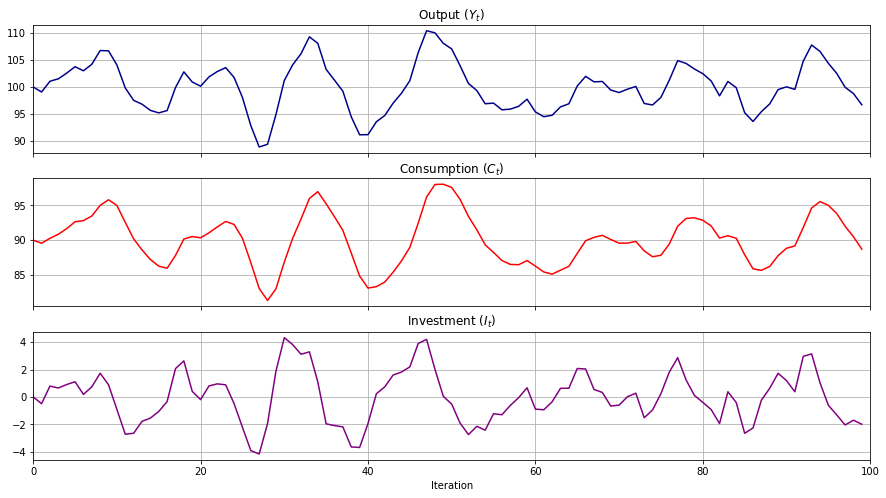In :
samlss.plot_simulation(100, stationary=True)
plt.show()

/anaconda3/lib/python3.6/site-packages/quantecon/lss.py:173: RuntimeWarning: covariance is not positive-semidefinite.
x0 = multivariate_normal(self.mu_0.flatten(), self.Sigma_0)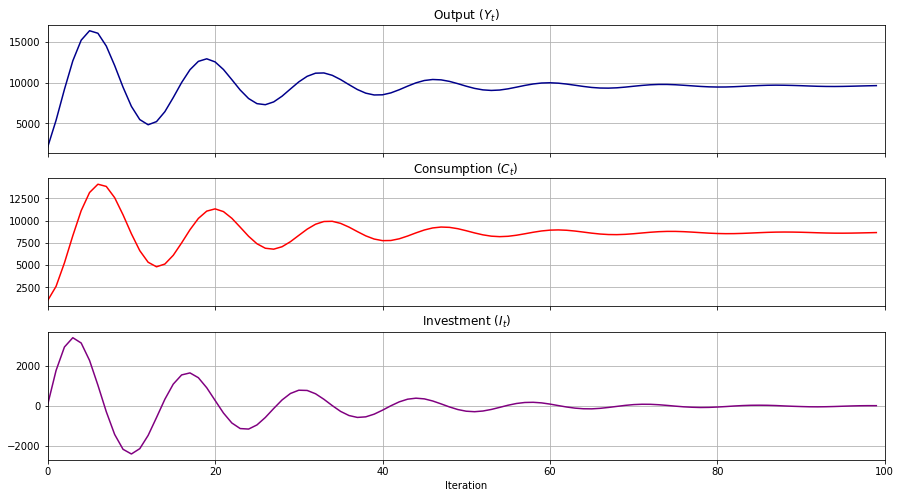In :
samlss.plot_irf(100)
plt.show()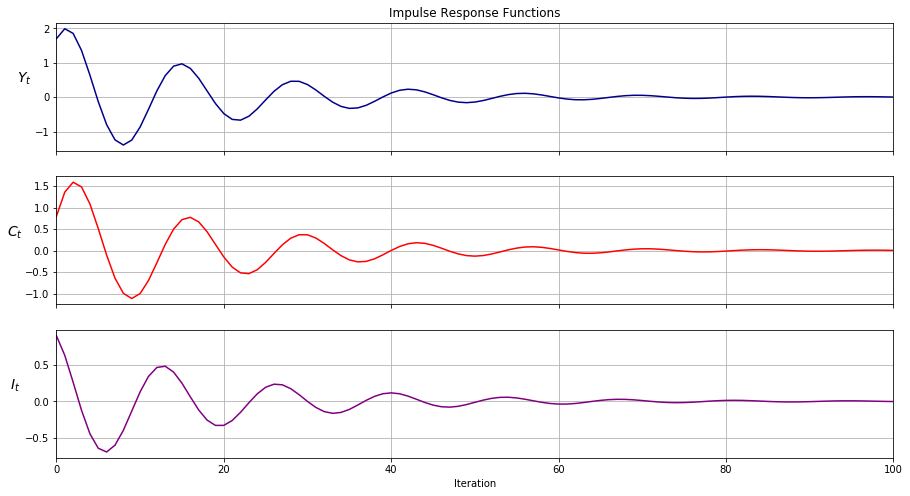In :
samlss.multipliers()

Out:
array([ 7.414389,  6.835896,  0.578493])
none
array([ 7.414389,  6.835896,  0.578493])

## Pure multiplier model¶

Let’s shut down the accelerator by setting $b=0$ to get a pure multiplier model

• the absence of cycles gives an idea about why Samuelson included the accelerator
In :
pure_multiplier = SamuelsonLSS(alpha=0.95, beta=0)

In :
pure_multiplier.plot_simulation()

Stationary distribution does not exist

Out: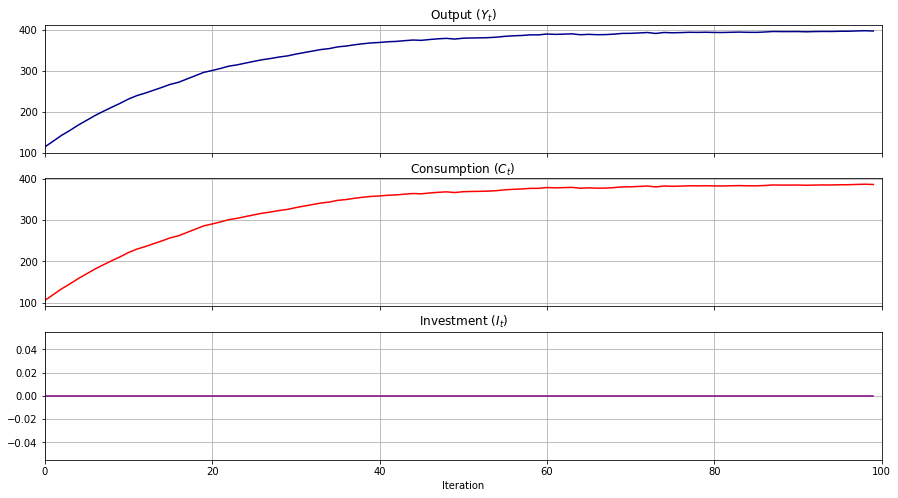none
Stationary distribution does not existIn :
pure_multiplier = SamuelsonLSS(alpha=0.8, beta=0)

In :
pure_multiplier.plot_simulation()

Out: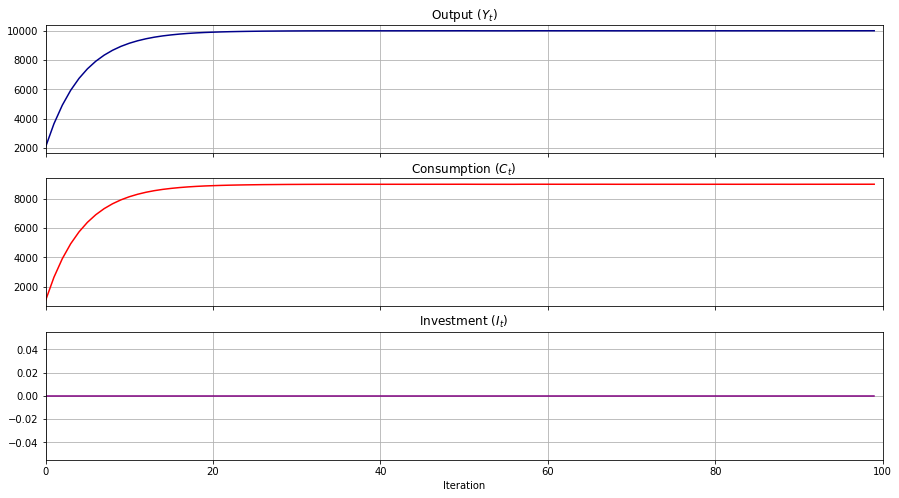In :
pure_multiplier.plot_irf(100)

Out: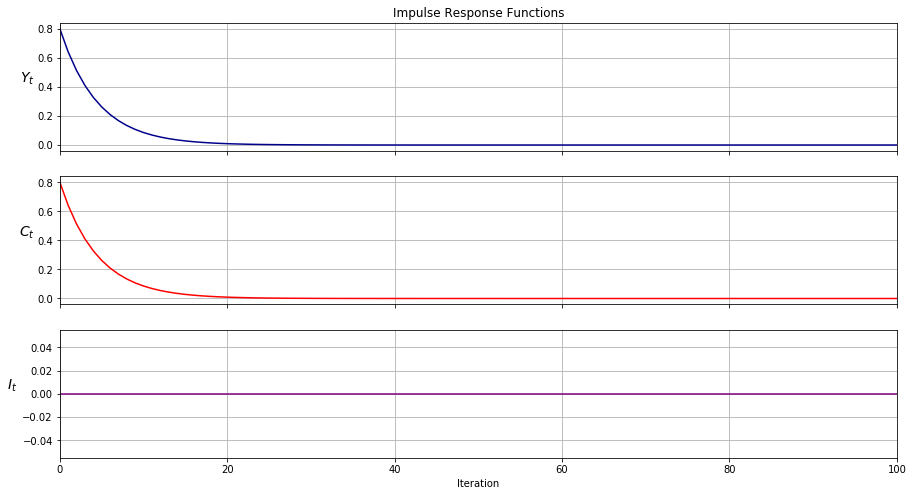## Summary¶

In this lecture, we wrote functions and classes to represent non-stochastic and stochastic versions of the Samuelson (1939) multiplier-accelerator model, described in [Sam39]

We saw that different parameter values led to different output paths, which could either be stationary, explosive, or ocillating

We also were able to represent the model using the QuantEcon.py LinearStateSpace class

 $$\left ( 2, \quad 6, \quad 1\right )$$